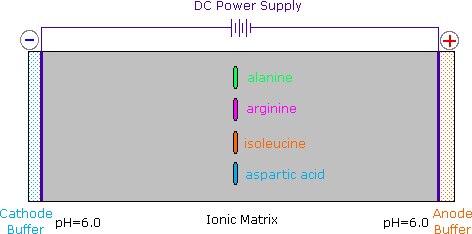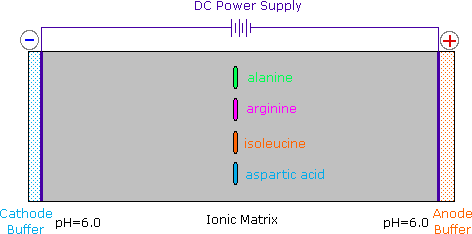# 26.2 Amino Acids, the Henderson-Hasselbalch Equation, and Isoelectric Points

Objectives

After completing this section, you should be able to

1. draw the predominant form of a given amino acid in a solution of known pH, given the isoelectric point of the amino acid.
2. describe, briefly, how a mixture of amino acids may be separated by paper electrophoresis.

Key Terms

Make certain that you can define, and use in context, the key terms below.

• electrophoresis
• isoelectric point

Since amino acids, as well as peptides and proteins, incorporate both acidic and basic functional groups, the predominant molecular species present in an aqueous solution will depend on the pH of the solution. In order to determine the nature of the molecular and ionic species that are present in aqueous solutions at different pH's, we make use of the Henderson-Hasselbalch Equation, written below. Here, the pKa represents the acidity of a specific conjugate acid function (HA). When the pH of the solution equals pKa, the concentrations of HA and A(-) must be equal (log 1 = 0).

$pK_a = pH + \log_{10} \dfrac{[HA]}{A^-]}$

The titration curve for alanine in Figure $$\PageIndex{2}$$ demonstrates this relationship. At a pH lower than 2, both the carboxylate and amine functions are protonated, so the alanine molecule has a net positive charge. At a pH greater than 10, the amine exists as a neutral base and the carboxyl as its conjugate base, so the alanine molecule has a net negative charge. At intermediate pH's the zwitterion concentration increases, and at a characteristic pH, called the isoelectric point (pI), the negatively and positively charged molecular species are present in equal concentration. This behavior is general for simple (difunctional) amino acids. Starting from a fully protonated state, the pKa's of the acidic functions range from 1.8 to 2.4 for -CO2H, and 8.8 to 9.7 for -NH3(+). The isoelectric points range from 5.5 to 6.2. Titration curves show the neutralization of these acids by added base, and the change in pH during the titration.Figure $$\PageIndex{1}$$: Titration curves for many other amino acids may be examined at a useful site provided by The University of Virginia in Charlottesville.

The distribution of charged species in a sample can be shown experimentally by observing the movement of solute molecules in an electric field, using the technique of electrophoresis (Figure $$\PageIndex{2}$$). For such experiments an ionic buffer solution is incorporated in a solid matrix layer, composed of paper or a crosslinked gelatin-like substance. A small amount of the amino acid, peptide or protein sample is placed near the center of the matrix strip and an electric potential is applied at the ends of the strip, as shown in the following diagram. The solid structure of the matrix retards the diffusion of the solute molecules, which will remain where they are inserted, unless acted upon by the electrostatic potential.Figure $$\PageIndex{2}$$: In the example shown here, four different amino acids are examined simultaneously in a pH 6.00 buffered medium. To see the result of this experiment, click on the illustration. Note that the colors in the display are only a convenient reference, since these amino acids are colorless.

At pH 6.00 alanine and isoleucine exist on average as neutral zwitterionic molecules, and are not influenced by the electric field. Arginine is a basic amino acid. Both base functions exist as "onium" conjugate acids in the pH 6.00 matrix. The solute molecules of arginine therefore carry an excess positive charge, and they move toward the cathode. The two carboxyl functions in aspartic acid are both ionized at pH 6.00, and the negatively charged solute molecules move toward the anode in the electric field. Structures for all these species are shown to the right of the display.It should be clear that the result of this experiment is critically dependent on the pH of the matrix buffer. If we were to repeat the electrophoresis of these compounds at a pH of 3.80, the aspartic acid would remain at its point of origin, and the other amino acids would move toward the cathode. Ignoring differences in molecular size and shape, the arginine would move twice as fast as the alanine and isoleucine because its solute molecules on average would carry a double positive charge.

As noted earlier, the titration curves of simple amino acids display two inflection points, one due to the strongly acidic carboxyl group (pKa1 = 1.8 to 2.4), and the other for the less acidic ammonium function (pKa2 = 8.8 to 9.7). For the 2º-amino acid proline, pKa2 is 10.6, reflecting the greater basicity of 2º-amines.

Table $$\PageIndex{1}$$: pKa Values of Polyfunctional Amino Acids
Amino Acid α-CO2H pKa1 α-NH3 pKa2 Side Chain pKa3 pI
Arginine 2.1 9.0 12.5 10.8
Aspartic Acid 2.1 9.8 3.9 3.0
Cysteine 1.7 10.4 8.3 5.0
Glutamic Acid 2.2 9.7 4.3 3.2
Histidine 1.8 9.2 6.0 7.6
Lysine 2.2 9.0 10.5 9.8
Tyrosine 2.2 9.1 10.1 5.7

Some amino acids have additional acidic or basic functions in their side chains. These compounds are listed in Table $$\PageIndex{1}$$. A third pKa, representing the acidity or basicity of the extra function, is listed in the fourth column of the table. The pI's of these amino acids (last column) are often very different from those noted above for the simpler members. As expected, such compounds display three inflection points in their titration curves, illustrated by the titrations of arginine and aspartic acid (Figure\ (\PageIndex{3}\)). For each of these compounds four possible charged species are possible, one of which has no overall charge. Formulas for these species are written to the right of the titration curves, together with the pH at which each is expected to predominate. The very high pH required to remove the last acidic proton from arginine reflects the exceptionally high basicity of the guanidine moiety at the end of the side chain.Figure $$\PageIndex{3}$$

## The Isoelectric Point

The isoelectric point, pI, is the pH of an aqueous solution of an amino acid (or peptide) at which the molecules on average have no net charge. In other words, the positively charged groups are exactly balanced by the negatively charged groups. For simple amino acids such as alanine, the pI is an average of the pKa's of the carboxyl (2.34) and ammonium (9.69) groups. Thus, the pI for alanine is calculated to be: (2.34 + 9.69)/2 = 6.02, the experimentally determined value. If additional acidic or basic groups are present as side-chain functions, the pI is the average of the pKa's of the two most similar acids. To assist in determining similarity we define two classes of acids. The first consists of acids that are neutral in their protonated form (e.g. CO2H & SH). The second includes acids that are positively charged in their protonated state (e.g. -NH3+). In the case of aspartic acid, the similar acids are the alpha-carboxyl function (pKa = 2.1) and the side-chain carboxyl function (pKa = 3.9), so pI = (2.1 + 3.9)/2 = 3.0. For arginine, the similar acids are the guanidinium species on the side-chain (pKa = 12.5) and the alpha-ammonium function (pKa = 9.0), so the calculated pI = (12.5 + 9.0)/2 = 10.75